EngliSea > M > math > LitionaryProverbs and Quotes in English
Short sentences and easy words.

◎◎◎ complement ⪢⪢
When two angles are complementary, we say that one angle is the complement of the other. #math
9593◎◎◎ complementary ⪢⪢
If two angles are complementary, then the sum of their measures is 90 degrees. ￫ measure #math
9684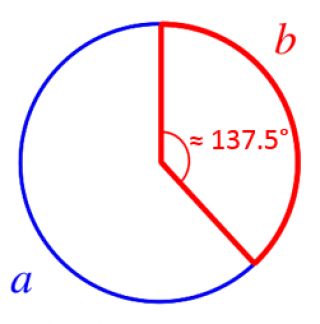◎◎◎ complex number ⪢⪢
A complex number is a number that can be expressed in the form a + bi where a and b are real numbers and i is the square root of -1. #math
9607

◎◎◎ congruent ⪢⪢
#math
3860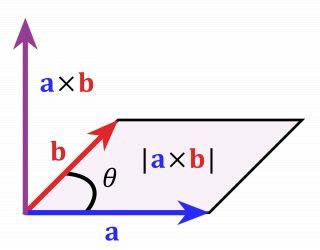Cross Product
Given two nonzero vectors in two or three dimensions, their cross product is a vector with magnitude equal to the product of the magnitudes of the vectors times the sine of the angle between the vectors and direction perpendicular to the vectors.
5291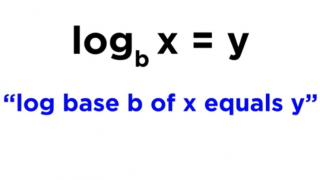○○○ Golden Section ⪢⪢
It was the Greek mathematician Euclid who produced the first precise description of the golden section. A length is devided into two parts in such a way that the smaller part is to the larger part in the same proportion as the larger one is to the whole.
1780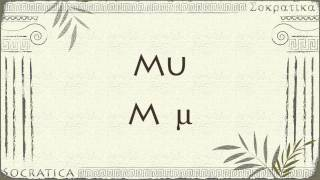Greek alphabet
Α α Β β Γ γ Δ δ Ε ε Ζ ζ Η η Θ θ Ι ι Κ κ Λ λ Μ μ Ν ν Ξ ξ Ο ο Π π Ρ ρ ϱ Σ σ/ς Τ τ Υ υ Φ φ Χ χ Ψ ψ Ω ω
5203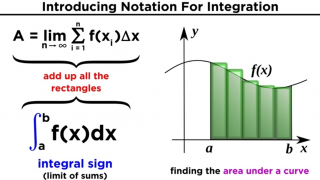◌◌◌ Integration
With the sum of f of x i times delta x from i equals one to infinity in the limit of n approaching infinity.
3658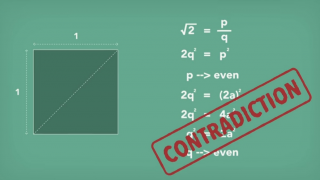irrational number
Irrational numbers can't be expressed as the ratio of two integers. #math
9591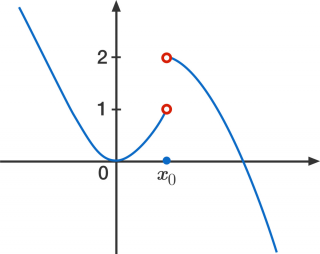◌◌◌ Limit
The limit of f of x as x approaches a is L.
3473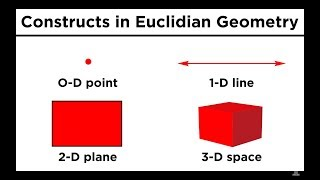◌◌◌ Line
From here, we can construct a one- dimensional object by stringing an infinite number of points along a particular dimension. This object is called a line.
5177

Linear systems
A collection of 2 or more linear equations
3810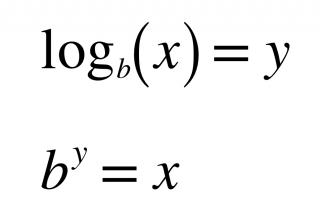○○○ logarithm ⪢⪢
What power do you have to raise b to in oder to get x? ❶ b˄y = x ❷ x˅y = b ❸ x⍻b = y #math
3784 COMMENT

○○○ logarithm ⪢⪢
x⍻b = y With logs, the base of the log raised to the power of what's on the other side of the equal sign will equal the number that the log is operating on. #math
6085 COMMENT

◌◌◌ loop
#math
16024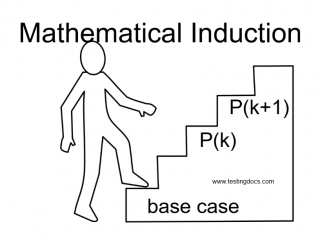Mathematical Induction
Let P(n) be a statement for each natural number n. If (a) P(1) is true, and (b) P(k) true ⇒ P(k+1) true for every natural number k∈ℕ then P(n) is true for all n∈ℕ.
9716

○○○ mold ⪢⪢
A sphere and a cube are topologically the same thing since you can just kind of mold one into the other. #math
16021◌◌◌ Plane
By stringing an infinite number of lines along a dimension perpendicular to the line, a two-dimensional object called a plane can be obtained. And then if we string an infinite number of planes in either direction, we get three dimensional space.
5031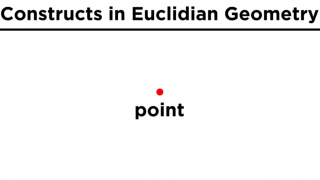◌◌◌ Point
First we look at a point. This is nothing more than a location in space. It is zero-dimensional, meaning that it has no dimensions of any kind.
5030

◌◌◌ Point
We represent points with little dots and some capital letter, making sure to realize that the dot we draw is infinitely larger than the point it is meant to represent.
6290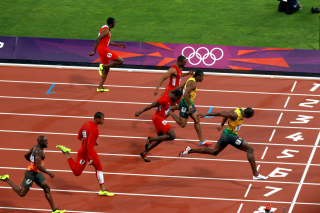power rule
(x˄n)ᐁx = n·x˄(n−1) #math
9686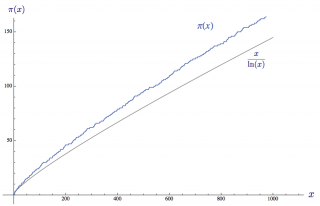prime number theorem
○ π(n) is asymptotically equivalent to x/log x. ○ Of the first n integers, roughly 1/log n of them would be prime. #math
9583

○○○ rhombus ⪢⪢
#pronunciation
20603◌◌◌ Space
By stringing an infinite number of lines along a dimension perpendicular to the line, a two-dimensional object called a plane can be obtained. And then if we string an infinite number of planes in either direction, we get three dimensional space.
5178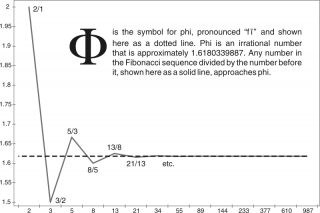Φ ≈ 1.618
Keplar observed that the relationship between a number in Fibonacci sequence and the previous number more and more closely approaches the irrational number Φ the longer the sequence is continued and Φ describes nothing other than the golden section. #math
9464○○○ ψ ≈ 137.5° ⪢⪢
Divides the angle of 360° in the proportions of the golden section. As angles smaller than 180° proved more handy in practice, the smaller of the resultant angles is usually called golden angle. #math
9465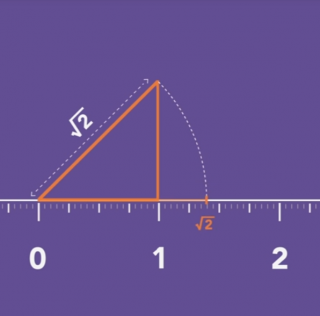√2 ≈ 1.414
According to Pythagoras theorem the diagonal length of a square with each side measuring one unit would be square root of two. The assumption that square root of two could be expressed as a ratio of two integers deduces a contradiction. #math
9592-